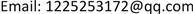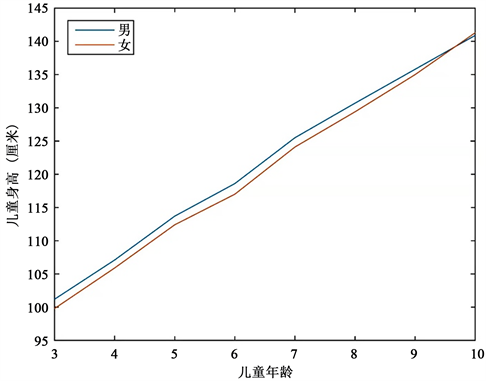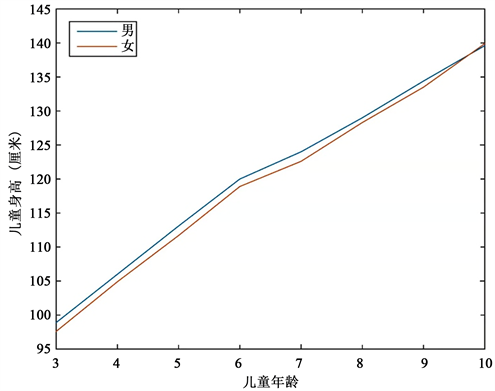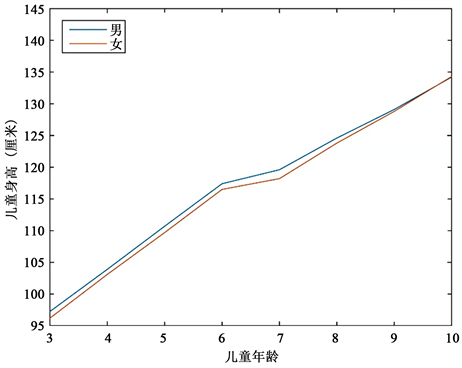﻿ 基于多元统计的儿童票定价策略的更新研究 A Study on Updating Children Ticket Pricing Strategies Based on Multivariate Statistics

Statistics and Application
Vol. 08  No. 06 ( 2019 ), Article ID: 33553 , 10 pages
10.12677/SA.2019.86106

A Study on Updating Children Ticket Pricing Strategies Based on Multivariate Statistics

Yulu Li, Zhiyuan Zheng, Yunqing Ma, Yang Shao

Shanghai University of International Business and Economics, ShanghaiReceived: Nov. 30th, 2019; accepted: Dec. 13th, 2019; published: Dec. 20th, 2019ABSTRACT

Nowadays, with the increasing living conditions of the people, more families will choose to travel to spend their leisure time. Therefore, the ticket pricing issues of tourist attractions, parks, etc. are gradually being discussed for everyone; The current height of children is generally higher than in the past and is controversial. The criteria for meeting the purchase of children’s tickets are key issues. In the past, the method of fixing 1.2 meters as the standard of height is no longer the most suitable standard for measurement under the conditions of different living standards, geographical factors and different levels of nutritional intake of children in various regions. Therefore, this paper attempts to test the height of children from different regions and different ages by using the profilometry method to collect the average height data of children aged 3 to 10 in the national, Shanghai, Jiangxi and Sichuan regions from 2000 to 2015. Whether the contours are similar, to determine whether it is necessary to set different height standards by region; to check whether the contours of children’s heights coincide in different eras, and to judge whether it is necessary to set new height standards today. Using MATLAB software to analyze the data and obtain the results, the results show that the height contours of each region are completely similar, so it is possible to develop uniform standards from all over the country; there are significant differences in heights in each era, so it is necessary to update the height standards of children's tickets in the contemporary era.

Keywords:Children Ticket, Multivariate Statistical Analysis, Profile Analysis, MATLAB1. 引言

1.1. 课题缘起

2018年1月份，曾有一位爸爸带着10岁女儿到上海迪士尼游玩，女儿因高达1米4被要求购买成人票，最终这让法官爸爸起诉了迪士尼 。这件事把儿童票问题带到了人们眼前，引起了热议，不少学者对儿童票的标准问题进行了研究，提出了他们的意见。儿童票的施行是本着对儿童权益保护的宗旨，却让部分儿童的权益受到损害。这样实际生活中的一件事，说明了儿童票收费标准亟待更新。

1.2. 研究现状

2005年中国青少年成年身高已赶上日本；城市中，7岁男、女孩童身高(反映早期发育)的平均值，以及全学龄段身高的平均值已赶上日本，但因乡村群体的相对滞后，整体上仍低于日本，而我国各群体体格发育潜力都很大  (季成叶，张欣，尹小俭等，2011)。学者王坤也曾指出：根据我国疾控部门统计的数据显示，早在2012年全国6岁城市男童平均身高已达到1.2米，12岁未成年人平均身高已超过1.5米 。本次项目需要在这些对儿童身高变化研究的基础上，联合儿童票的制定进一步对儿童票标准加以改善。

1.3. 研究原因

2. 数据描述Figure 1. 2010 national 3 - 10 year old male and female children height line chartFigure 2. Broken line of height of children aged 3 - 10 in cities and towns in China in 2015Figure 3. Broken line of height of children aged 3 - 10 in rural areas of China in 2015

3. 两总体的轮廓分析

${H}_{01}:\left(\begin{array}{c}{\mu }_{12}-{\mu }_{11}\\ {\mu }_{13}-{\mu }_{12}\\ ⋮\\ {\mu }_{1p}-{\mu }_{1,p-1}\end{array}\right)=\left(\begin{array}{c}{\mu }_{22}-{\mu }_{21}\\ {\mu }_{23}-{\mu }_{22}\\ ⋮\\ {\mu }_{2p}-{\mu }_{2,p-1}\end{array}\right)$

${H}_{01}:C{\mu }_{1}=C{\mu }_{2},\text{\hspace{0.17em}}\text{\hspace{0.17em}}{H}_{02}:C{\mu }_{1}\ne C{\mu }_{2}$

$C=\left(\begin{array}{ccccc}-1& 1& 0& \cdots & 0\\ 0& -1& 1& \cdots & 0\\ ⋮& ⋮& ⋮& \ddots & ⋮\\ 0& 0& 0& \cdots & 1\end{array}\right)$

$\text{rank}\left(C\right)=p-1$

${T}^{2}=\frac{{n}_{1}{n}_{2}}{{n}_{1}\text{+}{n}_{2}}{\left(\stackrel{¯}{x}-\stackrel{¯}{y}\right)}^{\prime }{C}^{\prime }{\left(C{S}_{p}{C}^{\prime }\right)}^{-1}C\left(\stackrel{¯}{x}-\stackrel{¯}{y}\right)$

${T}^{2}\ge {T}_{\alpha }^{2}\left(p-1,{n}_{1}+{n}_{2}-2\right)$，则拒绝 ${H}_{01}$

${T}_{\alpha }^{2}\left(p-1,{n}_{1}+{n}_{2}-2\right)=\frac{\left(p-1\right)\left({n}_{1}+{n}_{2}-2\right)}{{n}_{1}+{n}_{2}-p}{F}_{\alpha }\left(p-1,{n}_{1}+{n}_{2}-p\right)$

${H}_{02}:\frac{{\mu }_{11}+{\mu }_{12}+\cdots +{\mu }_{1p}}{p}=\frac{{\mu }_{21}+{\mu }_{22}+\cdots +{\mu }_{2p}}{p}$

${H}_{02}:{1}^{\prime }{\mu }_{1}={1}^{\prime }{\mu }_{2},\text{\hspace{0.17em}}\text{\hspace{0.17em}}{H}_{12}:{1}^{\prime }{\mu }_{1}\ne {1}^{\prime }{\mu }_{2}$

${T}^{2}=\frac{{n}_{1}{n}_{2}}{{n}_{1}+{n}_{2}}{\left(\stackrel{¯}{x}-\stackrel{¯}{y}\right)}^{\prime }1{\left({1}^{\prime }{S}_{p}1\right)}^{-1}{1}^{\prime }\left(\stackrel{¯}{x}-\stackrel{¯}{y}\right)=\frac{{n}_{1}{n}_{2}}{{n}_{1}+{n}_{2}}\frac{\left[{1}^{\prime }\left(\stackrel{¯}{x}-\stackrel{¯}{y}\right)\right]}{{1}^{\prime }{S}_{p}1}$

$t=\frac{{1}^{\prime }\left(\stackrel{¯}{x}-\stackrel{¯}{y}\right)}{\sqrt{\left(\frac{1}{{n}_{1}}+\frac{1}{{n}_{2}}\right){1}^{\prime }{S}_{p}1}}$

${T}^{2}\ge {F}_{\alpha }\left(1,{n}_{1}+{n}_{2}-2\right)$，则拒绝 ${H}_{02}$

$|t|\ge {t}_{\alpha /2}\left({n}_{1}+{n}_{2}-2\right)$，则拒绝 ${H}_{02}$

4. 数据代入Table 2. Test statistics for different regions in hypothesis 1Table 3. Test statistics of different years in hypothesis 1

5. 数据分析

${T}^{2}\ge {T}_{\alpha }^{2}\left(p-1,{n}_{1}+{n}_{2}-2\right)$，则拒绝 ${H}_{01}$

${T}^{2}\ge {F}_{\alpha }\left(1,{n}_{1}+{n}_{2}-2\right)$，则拒绝 ${H}_{02}$

6. 结论

7. 不足与展望

A Study on Updating Children Ticket Pricing Strategies Based on Multivariate Statistics[J]. 统计学与应用, 2019, 08(06): 937-946. https://doi.org/10.12677/SA.2019.86106

1. 1. 王琨. 儿童票标准亟待变革[N]. 民生与法制时报, 2019-02-28(007).

2. 2. 佚名. 10岁女儿达1米4被要求买成人票法官爸爸起诉上海迪士尼：以身高为标准不公平[J/OL]. http://wemedia.ifeng.com/69604579/wemedia.shtml, 2018-07-17/2019-10-09.

3. 3. 潘铎印. 按年龄收取儿童票合理合法[N]. 中国旅游报, 2018-05-30(003).

4. 4. 季成叶, 张欣, 尹小俭, 等. 中日两国学生身高发育水平及发展趋势比较[J]. 中国学校卫生, 2011, 32(10): 1168-1173.

5. 5. 江德斌. 儿童票从“量身高”转为“看年龄”[N]. 中国审计报, 2019-07-22(007).

6. 6. 冯海宁. 儿童免票要兼顾身高和年龄[N]. 中国青年报, 2018(02).

7. 7. 王姿欢, 蒋竞雄, 杨琦, 等. 中国部分城市学龄前儿童身高生长状况研究[J]. 中国儿童保健杂志, 2016(10): 1028-1031.

8. 8. 史洪举. 买不买儿童票不能光看身高[N]. 江西日报, 2019(10).

9. 9. 徐建辉. 迪士尼儿童票不该双重标准[N]. 湖北日报, 2018-07-12(007).

10. 10. 丁家发. “身高兼顾年龄”当成儿童票标配[N]. 海口日报, 2019-09-23(006).

11. 11. 佚名. 上海市2005年国民体质监测公报[R]. https://max.book118.com/html/2015/0313/13270800.shtm, 2005/2019-10-09.

12. 12. 佚名. 2010年国民体质监测公报[R]. http://www.sport.gov.cn/n4/n145/c328627/content.html, 2011-09-02/2019-10-09.